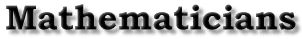Life | Work | Viete's construction of the regular heptagon | Bibliography | Back to the front page Viete's construction of the regular heptagon Robin Hartshorne Vieta is best known for his work in algebra. However, I would like to describe here some of his work in geometry, which seems to be less well known: the trisection of the angle, the solution of the ``casus irreducibilis'' of the cubic equation, and the construction of the regular heptagon. These results are all presented in the Supplementum Geometriae, first published in 1593 (though I have not been able to locate any copy of the original), and included in [Opera, 1646, pp. 240-257]. Vieta makes clear from the beginning that besides ruler and compass, he is admitting another operation: In order to supplement the defect of geometry, let it be allowed from any point to any two straight lines, to draw a straight line cutting off between them any segment fixed in advance. [Opera, p. 240] In other words, given two lines l,m, given a point A, and a segment d, we can draw a line through A so that the two lines l,m cut off on it a segment BC=d. Vieta also allows the same where a line and a circle are given instead of two lines. One can imagine this construction using a marked ruler: one makes two marks on the ruler at a distance d apart, then slides the ruler so that the marks lie over the lines l,m while passing still through the point A. This process goes by the name of neusis in Greek. Vieta does not give it a name. Vieta's work has 25 propositions, most of which I will skip over. Proposition IX [Opera, p. 245] is to trisect an angle. Let the given angle DBE be placed in a circle with B at the center. Draw a line through E, meeting BD extended at F, so that the segment FG cut off by the line and the circle is equal to BD (this is the neusis step). Then the angle at F is one third of the original angle at B. For the proof, draw BG. Call the angle at F 1. Then BFG is isosceles, so the angle GBF is also 1. The angle BGE is an exterior angle, hence equal to 2 times 1. BGE is isosceles, so GEB= 2. Now the original angle EBD is exterior to the triangle BEF so it is 3 times the angle at F. The question naturally arises, did Vieta discover this result himself, of did he learn it from earlier sources? He does not say. (In fact, contrary to the rules of scholarship today, by which one must scrupulously indicate sources and give credit where it is due, it seems to have been the custom in those days not to indicate sources. For example, Descartes in Book III of his Géométrie explains how any cubic or quartic equation can be solved by taking square roots, cube roots, or trisecting an angle: this result is Prop. XXV of Vieta's Supplementum Geometriae , but Descartes states it without giving any credit to Vieta. ) This same trisection appears in the Mathematical Collection of Pappus [....] and in the book of lemmas attributed to Archimedes [Archimedes, 1792, Prop VIII p. 358]. Now the latter is known only through an Arabic source, and was not published until 1657 [,,,], so we may assume that Vieta was unaware of it. However, Pappus' Mathematical Collection was circulating in manuscript in Europe well before the time of Vieta, and was published in a Latin translation by Commandino in 1588 [see intro. to Pappus, 1986], so we may presume that Vieta was familiar with the works of Pappus, and learned this construction from his book. (Commentators have also assumed that Vieta borrowed the terms zetetics and poristics from Pappus, Book VII-see [Viète , 1983, p. 11, note]). Proposition XVI [Opera, p. 248] gives in geometrical form the solution of a cubic equation using the trisection of an angle. The statement is as follows: If two isosceles triangles have their sides equal, and the angle at the base of the second is triple the angle at the base of the first, then the cube of the base of the first minus triple the solid made by the base of the first and the square of the common side, is equal to the solid on the base of the second and the same square of the common side. cm Let the first triangle be ABC with AB=BC, and the second CDE with CD=DE=BC, and assume that the angle DCE at the base of the second is three times the angle at A. Then he says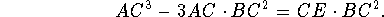Or, if we take the radius BC=1, let AC=x and CE=b, the equation is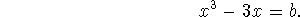Because the angle at A is one third of the angle DCE, the points A,B,D are collinear and we can use the previous diagram. Vieta's proof is expressed all in words. Translated into symbols it goes like this: drop perpendiculars from B and D to ACE, and let y=BD. By Euclid (III.36) we have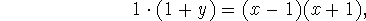using the point A and the two secants. Because the perpendiculars from B and D to ACE are parallel, we have a proportion (VI.2)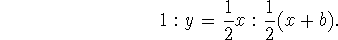Cross multiplying,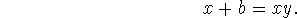Now, solving the first equation for y and substituting, we find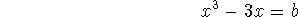as required. From the proposition of Vieta, we see that to solve the equation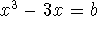, we make an isosceles triangle with sides 1 and base b, trisect the angle, and then the base of the new isosceles triangle will be x. By suitable linear substitutions, this gives a recipe for solving any cubic equation with three real roots, the so-called ``casus irreducibilis''. This is the case in which Cardano's formulas require cube roots of imaginary quantities, which were not understood at the time. As far as I know, this result is original to Vieta, and this is the place where he first explains it. Vieta gives the general rule for this case of the cubic equation in De Recognitione Equationum [Opera p.91], but without proof, referring to his book Theoremata ad sectiones angulares [Opera pp 287-304] for explanation. Now we come to the construction of the regular heptagon. Vieta's solution is directly analogous to Euclid's construction of the pentagon. Euclid first divides a line AB in extreme and mean ratio: that is he finds a point C for which AB:AC=AC:BC. (II.11). From this he constructs an isosceles triangle whose base angles are twice the angle at the vertex (IV.10). Then from this triangle he constructs the pentagon (IV.11). Vieta first extends the diameter BAC of a circle by a point I so that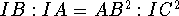(Prop. XIX). Form this he constructs an isosceles triangle whose base angles are three times the vertex angle (Prop XXIII), with which he can construct the heptagon (Prop XXIV). Here is the construction of I. Let the diameter of the circle be BAC, and call the radius 1. Take D with AD=(1/3). Let E be a vertex of the hexagon inscribed in the circle, so that CE is 1/6 of the circle. Draw DE. Make AF parallel to DE. Draw FGH so that GH=AB (neusis, trisecting the angle FAC). Draw EI parallel to FG. Then I is the required point. For the proof, draw AH. Make DK parallel to AH. Take L= midpoint of DC, and M= midpoint of DL. Then the triangles IKD and DEL satisfy the hypotheses of the previous result. So, if we let x=ID, r=DE and note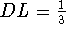,then x will satisfy the equation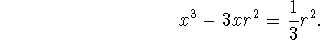Now apply (I.47) to the triangle DEM. The base isand the height is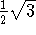, since ACE is an equilateral triangle. Thus we find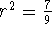, so x satisfies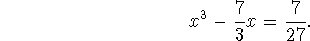To verify the relation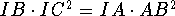, just note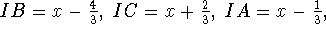and AB=1. Substituting gives the equation above, so I has the required property. Now, changing notation, let BAC be the diameter of a circle, let D be the point I as above, and draw DE=AB=1. Let DE cut the circle again at F, and draw AF. Then I claim BE is the side of the regular heptagon inscribed in the circle. Draw EC. By (III.36),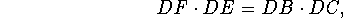but also by construction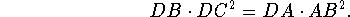Since DE=1=AB, we find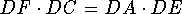. As a proportion, this says DF:DE=DA:DC. It follows that EC is parallel to AF (VI.2). Hence the angle at C is equal to the angle at BAF. Denote it by 1. On the other hand, the angle at C subtends the arc BE, so the angle at the center BAE is 2. So angle EAF is equal to 1, and we find AF is the angle bisector of BAE. Since DAE is isosceles, the angle at D is 2, and the exterior angle of the triangle DAF at F is 3. But AEF is isosceles, so the angle at AEF is also 3. Now we have an isosceles triangle AEF whose base angles are three times the vertex angle. It follows the vertex angle 1 is one seventh of two right angles. The angle BAE is twice that, so BE is the side of the regular heptagon, as required. Note. In this account I have shortened Vieta's exposition considerably by using modern algebraic notation, and in a few places I have done the calculation slightly differently, but in no way have I altered the basic mathematical content of the proof. History of constructions of the heptagon. There is an Arabic text, discovered only in 1927 containing a construction of the heptagon attributed to Archimedes, which depends on the following: Given a square ABCD with its diagonal AD, find a line CE so that the triangles CFD and BGE have the same area (see [van der Waerden, p.226] or [Knorr, p. 178ff]). This construction could be effected with an intersection of two conics, but it is not clear how to do it with a neusis. Campanus, in his commentary to Euclid, Book IV, mentions that you could construct a heptagon if you had an isosceles triangle whose base angles are three times the vertex angle. [Euclid 1516, f 56 verso] Otherwise, this construction of the heptagon seems to be totally original to Vieta. From a modern point of view, to construct a heptagon one must solve the cyclotomic equation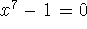. If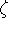is a root, then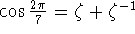is a root of the cubic equation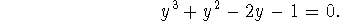One knows (from Vieta!) that such a cubic can be solved by trisecting a certain angle, and modern constructions have been given following this idea by [Plemelj, 1912], [Bieberbach, 1952], [Gleason, 1988], and [Hartshorne, 1997]. But none of these authors seems to have been aware of Vieta's construction, almost 400 years earlier, based on the same ideas, but without the benefit of the modern theory of the cyclotomic equation and complex numbers. Life | Work | Viete's construction of the regular heptagon | Bibliography | Back to the front page